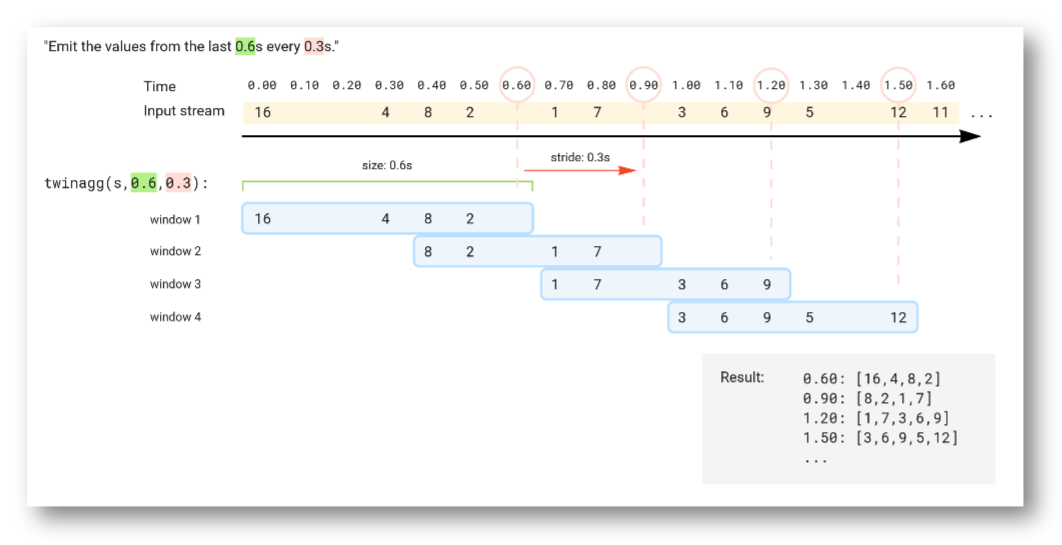# OSQL Essentials

This is an interactive guide using SA Engine for Wasm. To learn more about what this means you can read the Interactive documentation! section.

A few things to think about when using Wasm guides:

1. 📋 Execute code blocks in order: Code blocks in interactive guides are often designed to be executed in the order they appear. So if you skip a codeblock subsequent codeblocks might fail due to missing dependencies.
2. 📝 Edit examples: You can edit the code in the code blocks if you you want to try changing the example.
3. 📈 Change Visualization: You can change how the output of a query is visualized by specifying //plot: <vis-format> at the top of the code block. Available visualization formats are described in the Visualization chapter of the SA Studio manual.
4. 🔃 Page reload clears state: The SA Engine instance is cleared every time the page is reloaded. So if you are partway through a guide or tutorial and reload the page, you have to execute the code blocks from the beginning again before you continue with the rest of the guide. Otherwise remaining codeblocks might fail due to missing dependencies.
Guide specification
Guide type:Wasm code
Requirements:None

## Type extent and the solution domain​

Every type t has an extent(t) being the set of all objects of type t. For example, the extent of the type Integer is "all integers from negative infinity to positive infinity", or $\{-\infty, ..., -2, -1, 0, 1, 2, ..., \infty\}$.

A select statement forms the Cartesian product of the extents of all variables in the from clause. The solution domain is the span of all extents. The where clause then sets conditions limiting the emitted result to a subset of the solution domain.

For example, consider the select statement below. It forms a Cartesian product of the extent of i, which is the set of all possible integers, with the extent of s, which is the set of all possible strings. The where clause has two conditions. One that limits i to the set $\{1,2,3\}$, and one that limits s to the set $\{"a","b","c"\}$. This restricts the result to the Cartesian product of

select i, s  from Integer i, Charstring s where i in bag(1,2,3)   and s in bag("a","b","c");

Running the above query gives the following result:

[1,"a"][1,"b"][1,"c"][2,"a"][2,"b"][2,"c"][3,"a"][3,"b"][3,"c"]

The figure below illustrates how the extents span the solution domain, and how the result is produced as the Cartesian product of the two extents limited by the conditions in the where clause: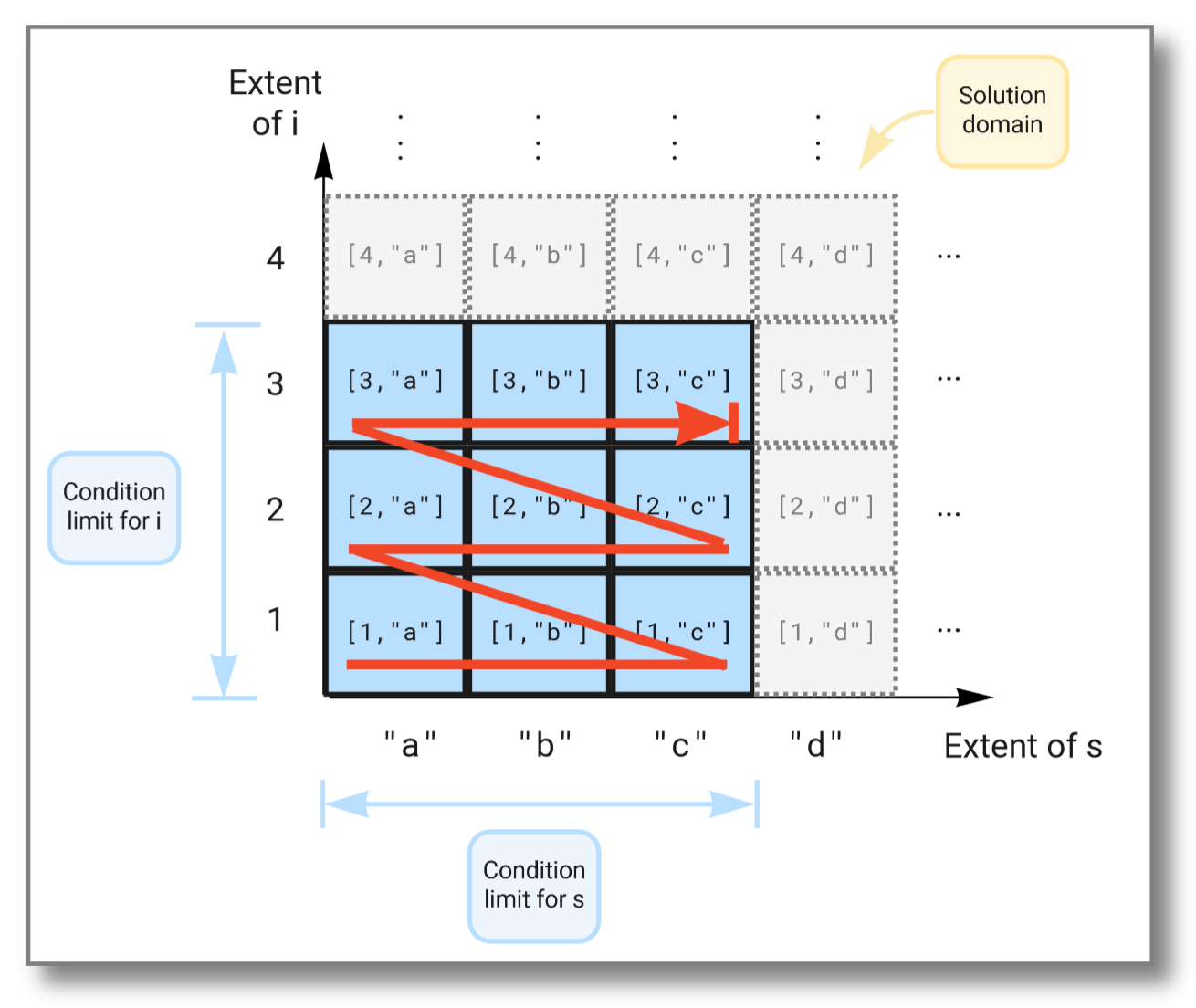We can limit the solution further by imposing additional conditions in the where clause, like this:

select i, s  from Integer i, Charstring s where i in bag(1,2,3)   and s in bag("a","b","c")   and i > 2;

Running the above query gives the following result:

[3,"a"][3,"b"][3,"c"]

This time the subset of the solution space is further limited due to the extra condition on i and now looks like this: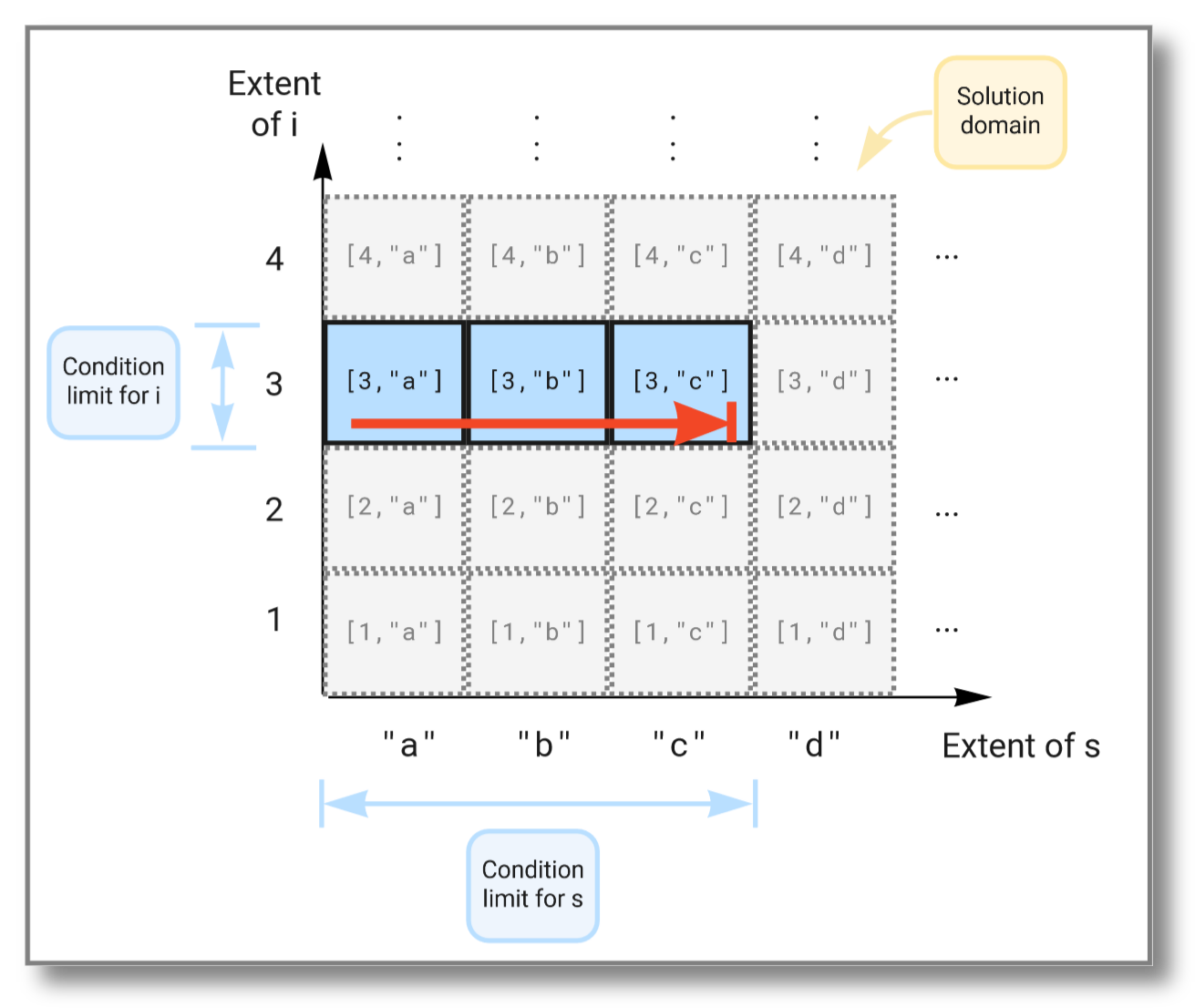Let's consider binding variables to infinite subsets in the solution domain. We can do this by using streams that never end. For example, heartbeat() generates a stream of seconds emitted at a given pace. This stream is infinite since time has no end. The select statement below tries to combine two such streams, but as we will see, taking the Cartesian product between two infinite sets will not work. Try running the query:

select s1, s2  from Number s1, Number s2 where s1 in heartbeat(1)   and s2 in heartbeat(1);

The result should be:

[0,0][0,1][0,2][0,3]...

We see that the first stream never increases because the second stream (which is evaluated first) never finishes. The figure below illustrates how the Cartesian product "travels" through the solution domain: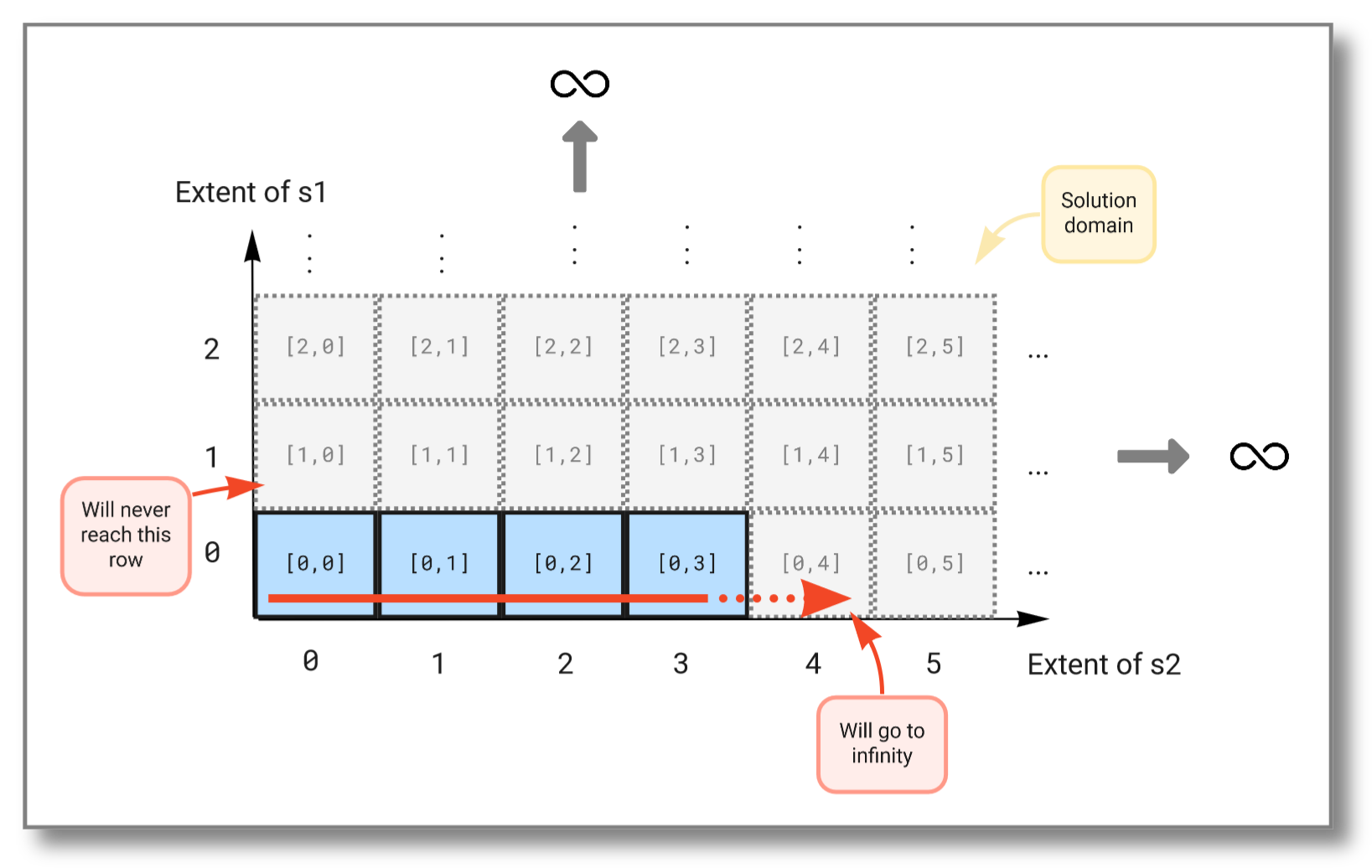To combine infinite streams you must instead use the streams:pivot() function. It combines the outputs of multiple streams into one vector and outputs a new vector each time one of the streams emits a result. The query below combines the streams from the previous example into a single stream:

select v  from Stream of Number s1, Stream of Number s2, Vector v where s1 = heartbeat(1)   and s2 = heartbeat(1)   and v in pivot([s1,s2]);

The result should be:

[0,null][0,0][1,0][1,1][2,1][2,2][3,2][3,3][4,3][4,4]...

As you see, this query will continue to infinity but include the values from both streams. If we look at the subset emitted from the solution domain, we see that the query "travels" diagonally through the solution domain: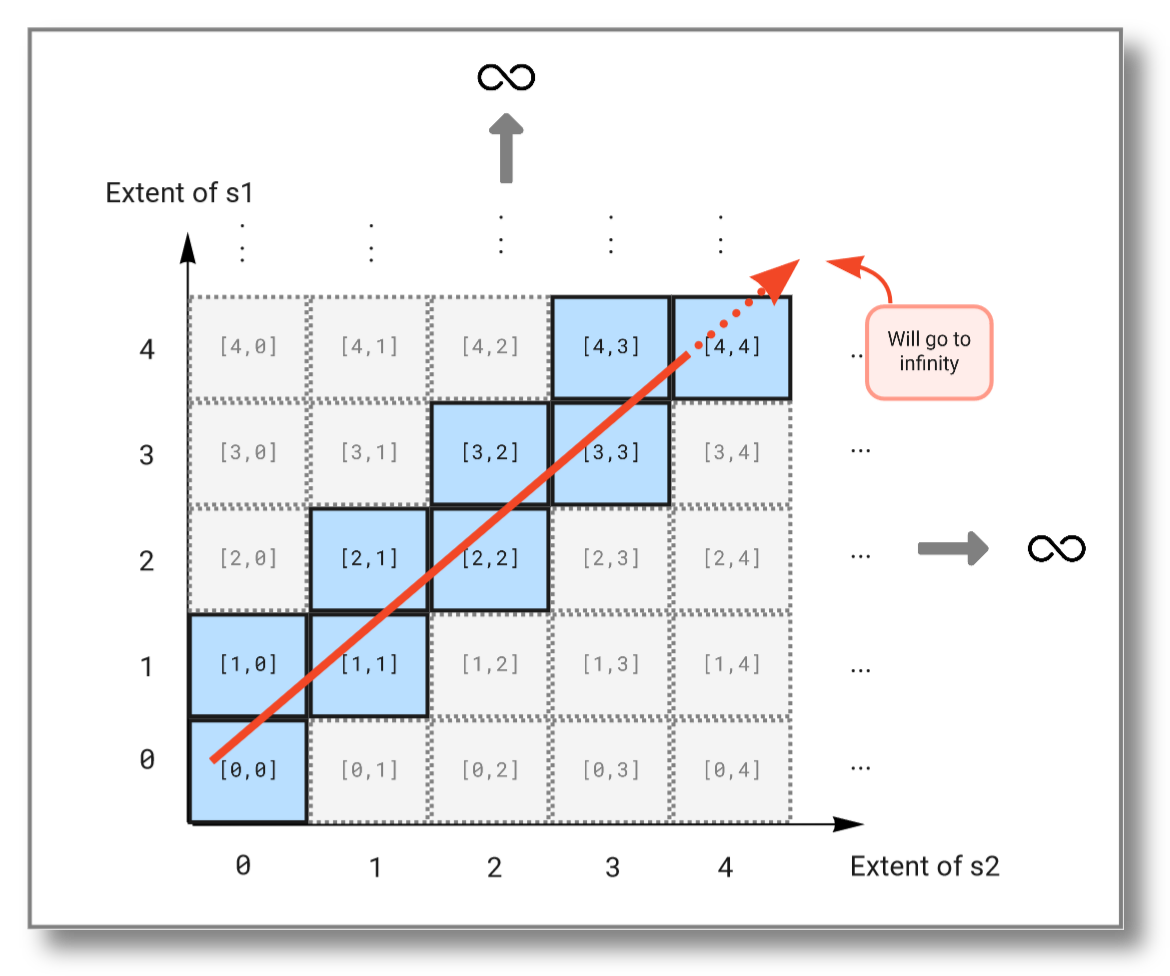## AND and OR and then SOME​

Select statements use AND clauses to join conditions that put contstraints on the output. Sometimes you want to have output that form disjoint components in the solution space. This is acheived by joining conditions with OR. However, in OSQL OR has to be used inside an AND clause with the keyword some.

For example, consider the following query which outputs the values of the vector [1,2,3,4,5] if the values are less than 3 OR larger than 4:

select i  from Integer i where i in [1,2,3,4,5]   and some(i<3 or i>4);

To get the negation of some we can use the keyword notany:

select i  from Integer i where i in [1,2,3,4,5]   and notany(i<3 or i>4);

SOME uses a return early pattern, so the entire expression within the SOME clause does not have to finish before SOME returns true.

For example, comparing the run times between

[sa.engine] 1> count(iota(1,10000000));100000000.328 s

and

[sa.engine] 1> some(iota(1,10000000));TRUE0.016 s

clearly shows that some exits on the first element while count has to step through all elements to determine the number of elements.

## Binding variables with "argmax"​

OSQL supports the argmax operation that finds the argument that gives the maximum value of a vector.

For example, the following query finds the index that gives the maximum of the vector [4,3,9,1,8,5]:

select i  from Integer i, Vector v where v = [4,3,9,1,8,5]   and v[i] = max(v);

Similarly you can use argmin to find the argument that gives the minimum value of a vector:

select i  from Integer i, Vector v where v = [4,3,9,1,8,5]   and v[i] = min(v);

Should there be more than one index that satifies the relation, then all of them are emitted:

select i  from Integer i, Vector v where v = [4,3,9,1,9,5]   and v[i] = max(v);

## Window functions​

SA Engine has two functions that make windows over streams. The first is called winagg() and is a count-based window function. The second function is called twinagg() and is a time-based window function.

### winagg()​

Winagg is a count-based window function that creates windows from an input stream. You specify the number of stream elements that each window contains (the window "size") and how many stream elements the window moves forward before emitting the next window (the "stride").

For example, to create a non-overlapping window (a tumbling window) you provide the same value for size and stride. Try it by running the following query (remember that heartbeat() generates a stream of seconds emitted at a given pace, so it takes 5s for the query to output any result):

winagg(heartbeat(1), 5, 5);

You can read the query as "emit the 5 latest elements every 5th element". The figure below illustrates the behavior of this query: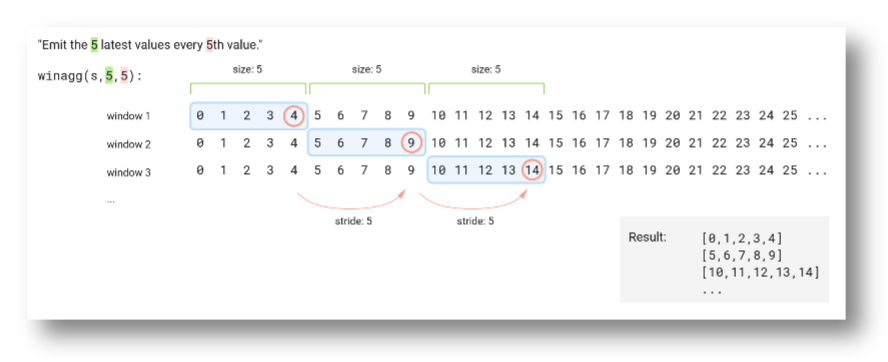It is not required to keep all the values from the input stream. You can specify a stride that is larger than the window size. Doing this will sample the stream every stride element. Try it by running the following query:

winagg(heartbeat(1), 4, 6);

You can read the query as "emit the 4 latest elements every 6th element". It effectively skips two values between every window, which is illustrated in the figure below: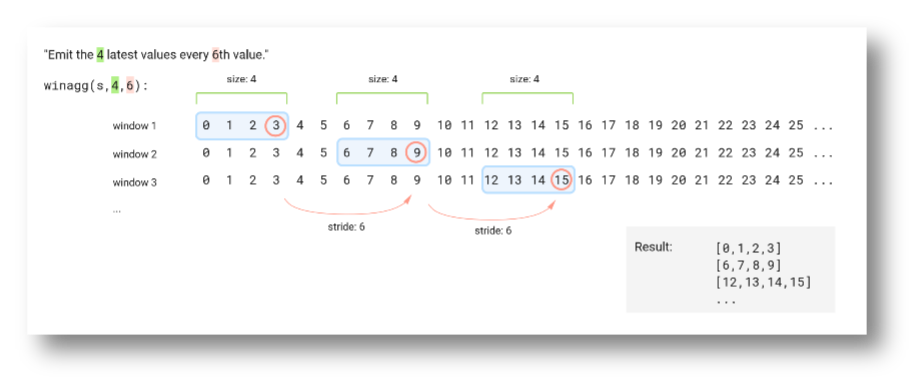If you set a stride value that is lower than the window size you get overlapping windows (sliding window). Try it by running the following query (it takes 10s for the query to output any result):

winagg(heartbeat(1), 10, 1);

The query produces a 10-element window every time the input stream emits a new element, which is illustrated in the figure below: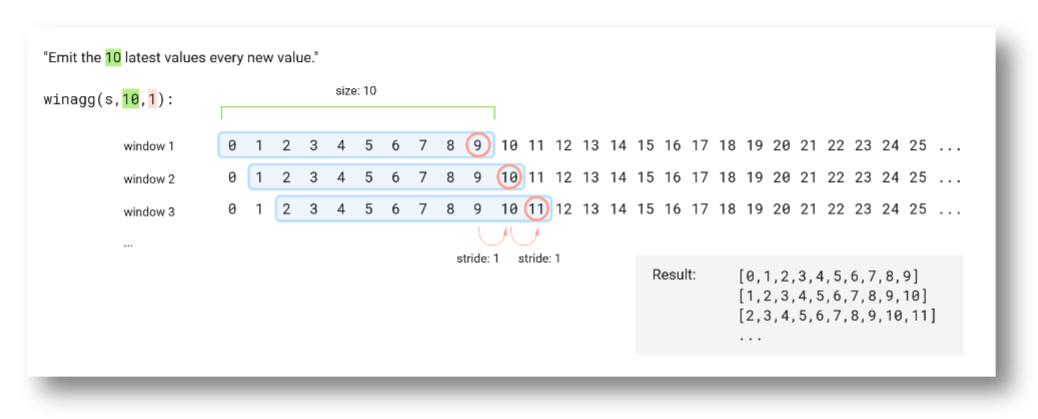### twinagg()​

Twinagg is a time-based window function that creates windows from an input stream of timestamped values. It works much like winagg but instead of specifying window size and stride in number of elements you specify it in seconds.

We will use a custom stream to illustrate how twinagg works. The function below outputs a stream of timestamped values. Create the function by running the query:

create function x() -> stream of timeval  as select stream of tt       from timeval tt      where tt in [ts(|2022-05-12T00:00:00.0Z|,16),                   ts(|2022-05-12T00:00:00.3Z|,4),                   ts(|2022-05-12T00:00:00.4Z|,8),                   ts(|2022-05-12T00:00:00.5Z|,2),                   ts(|2022-05-12T00:00:00.7Z|,1),                   ts(|2022-05-12T00:00:00.8Z|,7),                   ts(|2022-05-12T00:00:01.0Z|,3),                   ts(|2022-05-12T00:00:01.1Z|,6),                   ts(|2022-05-12T00:00:01.2Z|,9),                   ts(|2022-05-12T00:00:01.3Z|,5),                   ts(|2022-05-12T00:00:01.5Z|,12),                   ts(|2022-05-12T00:00:01.6Z|,11)];

If we illustrate the value stream on a timeline it looks like this: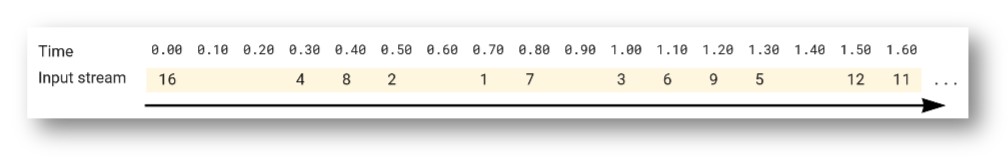To create a non-overlapping time window (a tumbling window) you provide the same value for size and stride. Try it by running the following query:

twinagg(x(), 0.3, 0.3);

The figure below illustrates how the tumbling window passes over the stream elements: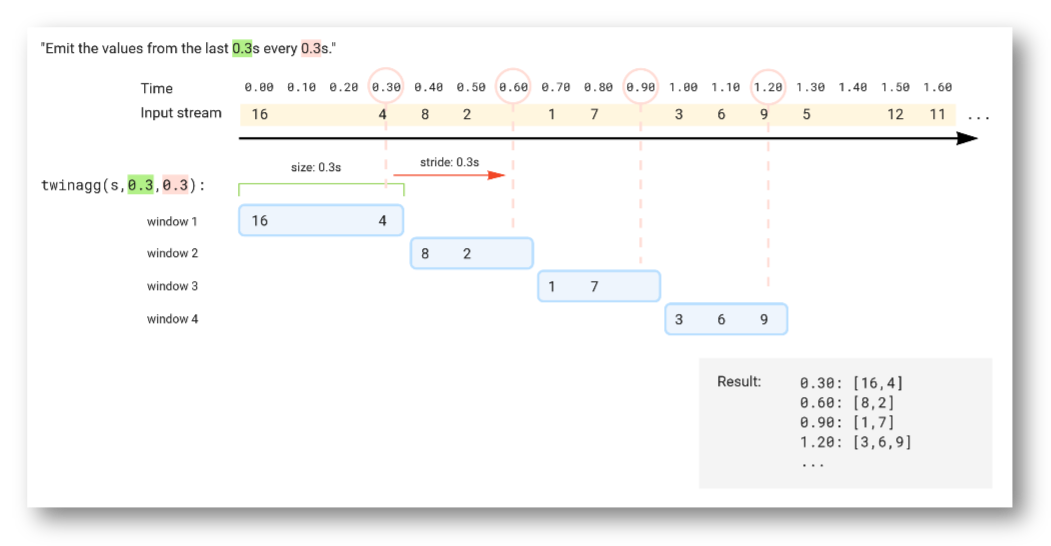Just like with winagg, twinagg can also skip elements by specifying a stride that is larger than the window size, or use a sliding window by specifying a stride that is smaller than the window size.

Here is an example where window size is larger than the stride. Try it by running the query:

twinagg(x(), 0.6, 0.3);

The query produces a 0.6 second window every 0.3 seconds, which is illustrated in the figure below: